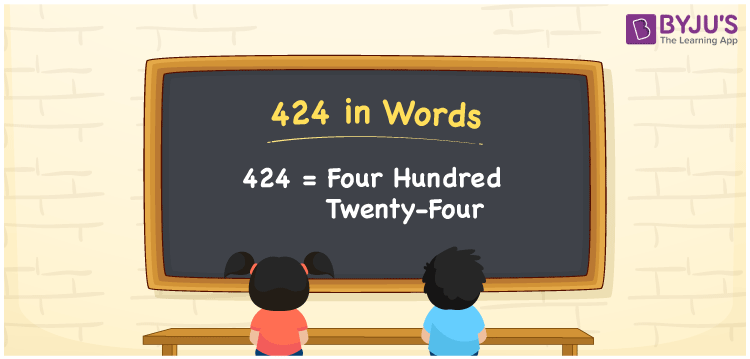# 424 in Words

424 in words can be written as Four Hundred Twenty-four. The concepts like counting numbers which are important from primary education are discussed here in brief. For instance, if you spend Rs. 424 in buying a cap, then you can say that “I bought a cap for Four Hundred Twenty-four Rupees”. The English alphabet is used to write 424 in words. Students can get a clear knowledge of numbers in words with the help of the study materials given at BYJU’S. The number 424 can be read as “Four Hundred Twenty-four” in English.

 424 in words Four Hundred Twenty-four Four Hundred Twenty-four in Numbers 424

## 424 in English Words## How to Write 424 in Words?

Place value chart and expanded form of 424 can be learn easily in this section. There are three digits in the number 424. The place value chart for 424 is provided below for reference to help students understand the concepts effectively.

 Hundreds Tens Ones 4 2 4

The expanded form of 424 is provided below:

4 × Hundred + 2 × Ten + 4 × One

= 4 × 100 + 2 × 10 + 4 × 1

= 424

= Four Hundred Twenty-four

Hence, 424 in words is written as Four Hundred Twenty-four.

424 is a natural number that precedes 425 and succeeds 423.

424 in words – Four Hundred Twenty-four

Is 424 an odd number? – No

Is 424 an even number? – Yes

Is 424 a perfect square number? – No

Is 424 a perfect cube number? – No

Is 424 a prime number? – No

Is 424 a composite number? – Yes

## Frequently Asked Questions on 424 in Words

Q1

### How can 424 be written in words?

424 can be written as “Four Hundred Twenty-four” in words.
Q2

### Is 424 an odd number?

No, 424 is an even number because it is divisible by 2.
Q3

### Write Four Hundred Twenty-four in numbers.

Four Hundred Twenty-four can be written in numbers as 424.